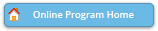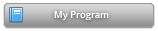#### Abstract Details

 Activity Number: 563 - Recent Advances in Bayesian Structure Learning Type: Topic Contributed Date/Time: Wednesday, July 31, 2019 : 2:00 PM to 3:50 PM Sponsor: Section on Bayesian Statistical Science Abstract #305329 Presentation Title: Bayesian Inference in Nonparanormal Graphical Models Author(s): Jami Mulgrave* and Subhashis Ghoshal Companies: and North Carolina State University Keywords: graphical model; rank likelihood; horseshoe prior; Bayesian regression; Gibbs sampling; nonparanormal Abstract: Gaussian graphical models, where it is assumed that the variables of interest jointly follow multivariate normal distributions with sparse precision matrices, have been used to study intrinsic dependence among several variables, but the Gaussianity assumption may be restrictive in many applications. A nonparanormal graphical model is a nonparametric generalization of a Gaussian graphical model for continuous variables where it is assumed that the variables follow a Gaussian graphical model only after some unknown smooth monotone transformation. We consider a Bayesian approach in the nonparanormal graphical model using a rank likelihood which remains invariant under monotone transformations, thereby avoiding the need to put a prior on the transformation functions. On the underlying precision matrix of the transformed variables, we consider a horseshoe prior using a Bayesian regression approach and use an efficient posterior Gibbs sampling scheme. We study the numerical performance of the proposed method through a simulation study and apply it on a real dataset.

Authors who are presenting talks have a * after their name.